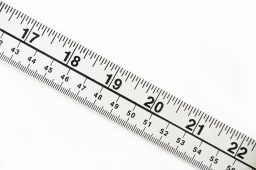Cutting wire

If you cut a 3 ½ ft length wire into pieces that are 2 inches long, how many pieces of wire will you have?

n =  21/1 = 21

Step-by-step explanation:Did you find an error or inaccuracy? Feel free to write us. Thank you!Tips to related online calculators
Need help to calculate sum, simplify or multiply fractions? Try our fraction calculator.
Do you want to convert length units?